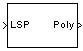# LSF/LSP to LPC Conversion

Convert line spectral frequencies or line spectral pairs to linear prediction coefficients

## Library

Estimation / Linear Prediction

`dsplp`

•## Description

The LSF/LSP to LPC Conversion block takes a vector or matrix of line spectral pairs (LSPs) or line spectral frequencies (LSFs) and converts it to a vector or matrix of linear prediction polynomial coefficients (LPCs). When converting LSFs to LPCs, the block outputs match those of the `lsf2poly` function.

The block input can be an N-by-M matrix or an unoriented vector. Each column of the matrix is treated as a channel. When the input is an unoriented vector, the input is treated as one channel. Each input channel must be in the same format, which you specify in the Input parameter:

• `LSF in range (0 pi)` — Vector of LSF values between 0 and π radians in increasing order. Do not include the guaranteed LSF values, 0 and π.

• `LSF normalized in range (0 0.5)` — Vector of normalized LSF values in increasing order, (compute by dividing the LSF values between 0 and π radians by 2π). Do not include the guaranteed normalized LSF values, 0 and 0.5.

• `LSP in range (-1 1)` — Vector of LSP values in decreasing order, equal to the cosine of the LSF values between 0 and π radians. Do not include the guaranteed LSP values, -1 and 1.

## Parameters

Input

Specifies whether to convert ```LSP in range (-1 1)```, `LSF in range (0 pi)`, or `LSF normalized in range (0 0.5)` to linear prediction coefficients (LPCs).

## Supported Data Types

• Double-precision floating point

• Single-precision floating point

## References

Kabal, P. and Ramachandran, R. “The Computation of Line Spectral Frequencies Using Chebyshev Polynomials.” IEEE Transactions on Acoustics, Speech, and Signal Processing, Vol. ASSP-34 No. 6, December 1986. pp. 1419-1426.

 LPC to LSF/LSP Conversion DSP System Toolbox LPC to/from RC DSP System Toolbox LPC/RC to Autocorrelation DSP System Toolbox `lsf2poly` Signal Processing Toolbox

## Extended Capabilities

### C/C++ Code GenerationGenerate C and C++ code using Simulink® Coder™.

Introduced before R2006a

## Support